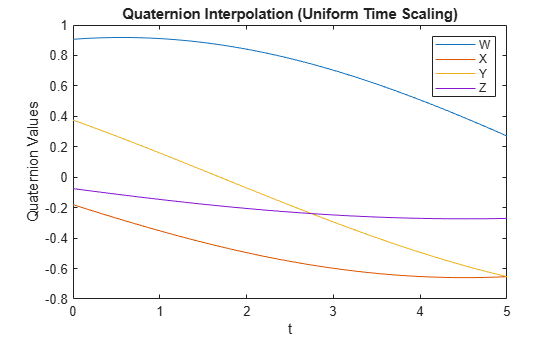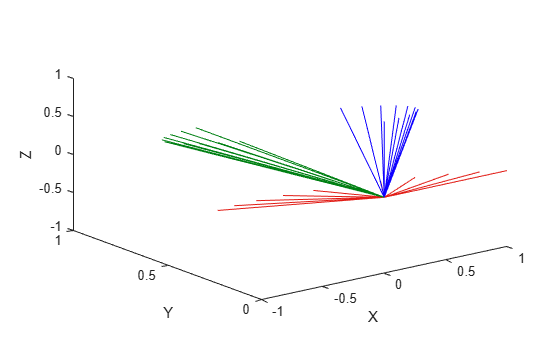rottraj

Generate trajectories between orientation rotation matrices

Description

example

[R,omega,alpha] = rottraj(r0,rF,tInterval,tSamples) generates a trajectory that interpolates between two orientations, r0 and rF, with points based on the time interval and given time samples.

[R,omega,alpha] = rottraj(r0,rF,tInterval,tSamples,Name,Value) specifies additional parameters using Name,Value pair arguments.

Examples

collapse all

Define two quaternion waypoints to interpolate between.

q0 = quaternion([0 pi/4 -pi/8],'euler','ZYX','point');
qF = quaternion([3*pi/2 0 -3*pi/4],'euler','ZYX','point');

Specify a vector of times to sample the quaternion trajectory.

tvec = 0:0.01:5;

Generate the trajectory. Plot the results.

[qInterp1,w1,a1] = rottraj(q0,qF,[0 5],tvec);

plot(tvec,compact(qInterp1))
title('Quaternion Interpolation (Uniform Time Scaling)')
xlabel('t')
ylabel('Quaternion Values')
legend('W','X','Y','Z')Define two rotation matrix waypoints to interpolate between.

r0 = [1 0 0; 0 1 0; 0 0 1];
rF = [0 0 1; 1 0 0; 0 0 0];

Specify a vector of times to sample the quaternion trajectory.

tvec = 0:0.1:1;

Generate the trajectory. Plot the results using plotTransforms. Convert the rotation matrices to quaternions and specify zero translation. The figure shows all the intermediate rotations of the coordinate frame.

[rInterp1,w1,a1] = rottraj(r0,rF,[0 1],tvec);

rotations = rotm2quat(rInterp1);
zeroVect = zeros(length(rotations),1);
translations = [zeroVect,zeroVect,zeroVect];

plotTransforms(translations,rotations)
xlabel('X')
ylabel('Y')
zlabel('Z')Input Arguments

collapse all

Initial orientation, specified as a 3-by-3 rotation matrix or quaternion object. The function generates a trajectory that starts at the initial orientation, r0, and goes to the final orientation, rF.

Example: quaternion([0 pi/4 -pi/8],'euler','ZYX','point');

Data Types: single | double

Final orientation, specified as a 3-by-3 rotation matrix or quaternion object. The function generates a trajectory that starts at the initial orientation, r0, and goes to the final orientation, rF.

Example: quaternion([3*pi/2 0 -3*pi/4],'euler','ZYX','point')

Data Types: single | double

Start and end times for the trajectory, specified as a two-element vector.

Example: [0 10]

Data Types: single | double

Time samples for the trajectory, specified as an m-element vector.

Example: 0:0.01:10

Data Types: single | double

Name-Value Arguments

Specify optional comma-separated pairs of Name,Value arguments. Name is the argument name and Value is the corresponding value. Name must appear inside quotes. You can specify several name and value pair arguments in any order as Name1,Value1,...,NameN,ValueN.

Example: 'TimeScaling',[0 1 2; 0 1 0; 0 0 0]

Time scaling vector and the first two derivatives, specified as the comma-separated pair of 'TimeScaling' and a 3-by-m vector, where m is the length of tSamples. By default, the time scaling is a linear time scaling between the time points in tInterval.

For a nonlinear time scaling, specify the values of the time points in the first row. The second and third rows are the velocity and acceleration of the time points, respectively. For example, to follow the path with a linear velocity to the halfway point, and then jump to the end, the time-scaling would be:

s(1,:) = [0 0.25 0.5 1 1 1] % Position
s(2,:) = [1    1   1 0 0 0] % Velocity
s(3,:) = [0    0   0 0 0 0] % Acceleration

Data Types: single | double

Output Arguments

collapse all

Orientation trajectory, returned as a 3-by-3-by-m rotation matrix array or quaternion object array, where m is the number of points in tSamples. The output type depends on the inputs from r0 and rF.

Orientation angular velocity, returned as a 3-by-m matrix, where m is the number of points in tSamples.

Orientation angular acceleration, returned as a 3-by-m matrix, where m is the number of points in tSamples

Limitations

• When specifying your r0 and rF input arguments as a 3-by-3 rotation matrix, they are converted to a quaternion object before interpolating the trajectory . If your rotation matrix does not follow a right-handed coordinate system or does not have a direct conversion to quaternions, this conversion may result in different initial and final rotations in the output trajectory.

 Dam, Erik B., Martin Koch, and Martin Lillholm. Quaternions, Interpolation and Animation. Technical Report DIKU-TR-98/5 (July 1998). http://web.mit.edu/2.998/www/QuaternionReport1.pdf

 Graf, Basile. Quaternions and Dynamics. arXiv:0811.2889 [math.DS] (2008). https://arxiv.org/pdf/0811.2889.pdf

 Lynch, Kevin M., and Frank C. Park. Modern Robotics: Mechanics, Planning, and Control. Cambridge University Press, 2017.